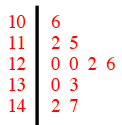### Home > MC2 > Chapter 5 > Lesson 5.2.3 > Problem5-70

5-70.

The girls' basketball team weighed each player. Their weights in pounds were $120$, $122$, $126$, $130$, $133$, $147$, $115$, $106$, $120$, $112$, and $142$. .

1. Make a stem-and-leaf plot of the teams' weights.

A stem-and-leaf plot displays data by ordering it least to greatest. All of the digits except for the last one form the stem and the last digit forms the leaf. The leaf portion is arranged in order from least to greatest.2. What is the median weight of girls on the team?

The median is the middle number in a set of data.

$122$

3. What is the range of the data?

Subtract the largest number by the smallest number. The range is the difference between the greatest and least number in a set of data.

4. What are the mean and mode?

The mean is the average of a set of numbers, while the mode is the number that occurs the most often in a set.

Explore the problem with the eTool below.
Click the link at right for the full version of the eTool: MC2 5-70 HW eTool